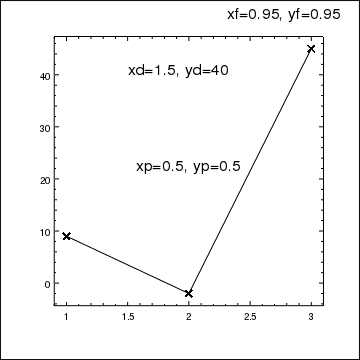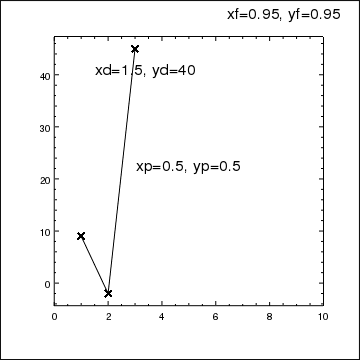## What coordinate system do I use for positioning labels?

The ChIPS GUI can be used to change both the location and the coordinate system of an annotation, such as a label.

Labels, as with many objects in ChIPS, can be created using one of the following coordinate systems:

1. data (the default, if axes have been created),
2. plot normalized,
3. frame normalized,
4. window normalized,
5. and pixel.

This allows labels to be positioned at a particular data coordinate (e.g. data coordinates of x=23.4, y=-0.04), at the top left of the plot (plot normalized coordinates of x=0.05, y=0.95), or at the bottom-right of a frame (frame normalized coordinates of x=0.95, y=0.05 with the halign attribute set to 1 so that the label is right justified). Once a label is created, its coordinate system can not be modified, although its location can be changed using move_label.

The data and plot-normalized systems are likely to be the main choices for placing a label; the former is useful when you want to label a feature and the latter for placing a label at a consistent location within a plot. One significant difference in the two systems is the behavior when the plot limits are changed; labels using the data coordinate system will be moved as the axis changes its display range whereas the plot-normalized coordinate system does not change when the axis limits change. The frame-normalized system is useful when adding labels outside the plot label (although for common cases such as plot title and axis labels it is often simpler to use the specialized versions).

In the code below we create a simple plot and then place labels using the data, plot normalized and frame normalized coordinate systems, and their behavior when changing axis limits is shown. The second label (placed using the plot-normalized system) is set to be centered horizontally at the given coordinate (halign=0.5) and the third label (frame normalized) is right-justified (halign=0.95).

chips> clear()
chips> set_frame(["border.visible", True])
chips> add_label(1.5, 40, "xd=1.5, yd=40", ["id", "data"])
chips> add_label(0.5, 0.5, "xp=0.5, yp=0.5", ["id", "plot", "coordsys", PLOT_NORM, "halign", 0.5])
chips> add_label(0.95, 0.95, "xf=0.95, yf=0.95", ["id", "frame", "coordsys", FRAME_NORM, "halign", 1])
chips> set_label('all', ['size', 20])At this point the data label is roughly half way across the plot, near the top, the plot label is in the middle of the plot and the frame label is in the top-right corner of the window, since the frame covers the window. The frame.visible attribute has been set to show the frame border, to indicate the edge of the frame in the PNG output included in this page.

chips> get_plot_xrange()
[0.9, 3.1]
chips> get_plot_yrange()
[-4.35, 47.35]
chips> limits(X_AXIS, 0, 10)Changing the X-axis limits has changed the relative location of the first label with respect to the plot (since x=1.5 is now nearer the left edge of the plot) but the other two labels have not moved.